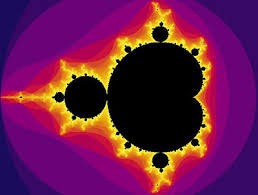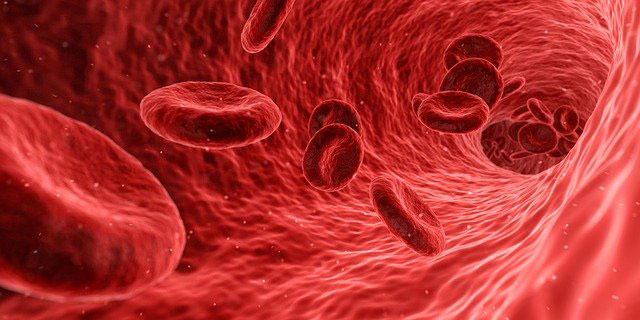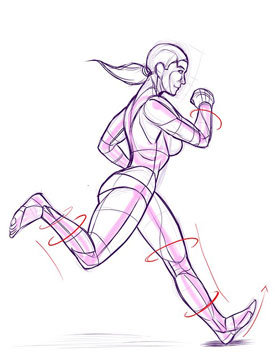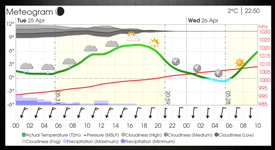# Three New Math Courses: Calculus I, Calculus II, and College AlgebraUNE Online is pleased to announce the development of three new math courses, Calculus I, Calculus II, and College Algebra, that are now available for registration.

Science Prerequisites for Health Professions (SPHP) courses are 100% online courses that are popular with students who need just a few college-level or post-baccalaureate-level science or math credits in order to apply for graduate school.

In general, SPHP classes are stand-alone courses designed to be taken one at a time. They are mostly intended for students who wish to enter a health professions program but may need one or two courses to fill a gap in their transcript.

SPHP students who successfully complete their prerequisite courses go on to apply to various health professions programs including Physician Assistant (PA), MD/DO, Nursing, Physical or Occupational Therapy, Veterinary, or other health professions graduate programs.

CGPS has developed three new online math courses in response to current student demand:

## Calculus I – MATH 1020Example project 1 from Calculus I: “Mathematics and Artistry Meet” in Iterative Processes and Chaos.
The concepts explored relate to population growth, thermal convection of fluids, dripping water faucets, and the firing of neurons in your brain. Students explore the mathematical construct of the Mandelbrot set, where they observe order arise from chaos. Students use math to create a Mandelbrot set like the one seen here.

Calculus I is a four-credit calculus course that focuses on single variable calculus through graphical, analytical, and numerical techniques. Differentiation and its applications are thoroughly discussed.

Basic integration techniques are introduced. Mathematical manipulation and computational competence are equally weighted with the ability to analyze, evaluate, synthesize, and form accurate decisions using relevant information in applied settings.

## Calculus I course outcomes include:

• Apply the core concepts of differential and integral calculus to solve problems in Calculus I:
• Limits and Continuity: Graphical interpretation, numerical approximation, limit laws, Squeeze Theorem, Intermediate Value Theorem, tangent and velocity problems, L’Hopital’s rule
• Derivatives: Formal definition of a derivative, Delta – Epsilon proofs, differentiation rules, trig formulas, chain, product and quotient rules, implicit and logarithmic differentiation
• Applications of the derivative: Rates of change, related rates, Mean Value Theorem. curve sketching, local and absolute extrema, optimization, linear approximations, Newton’s method.For the Grand Canyon skywalk project, students calculate the center of mass of the skywalk, and calculate how that changes when visitors walk out on it. Essentially, students apply math concepts to show how this phenomenon works.

• Integrals: Approximating areas, antidifferentiation, Riemann sums, Fundamental Theorem of Calculus, definite and indefinite integrals, substitution methods
• Applications of Integration: Area under and between curves, volumes of revolutions, arc length, work, hydrostatic force, moments and centers of mass, exponential growth and decay models, hyperbolic functions
• Utilize numerical, graphical, analytical, and approximation models in pure and applied settings.
• Develop visual literacy of mathematical concepts through graphical analysis.
• Communicate mathematical concepts and apply complex symbolic representation in written, verbal, and technological settings.
• Develop the ability to identify and apply multiple mathematical problem-solving techniques for a specific situation.
• Gain introductory level knowledge appropriate to a single variable calculus course of mathematical definitions and proofs of key theorems

Calculus I is a four-credit, lecture-only course. One semester of college-level pre-calculus is highly suggested as it supports course preparedness and success. We recommend having completed the listed prerequisites before enrolling and within the past seven years.

## Calculus II – MATH 1021Example project from Calculus II: Apply knowledge of differential equations and integration to imagine what researchers may do in order to model a disease outbreak. Use different strategies to model the outbreak of a disease in a local population and focus on the SIR model: Susceptible, Infected, and Recovered.

Calculus II is a four-credit calculus course that continues the study of calculus. More techniques of integration will be investigated in this course. The concepts of limits, differentiation, and integration will be applied to problems in differential equations, sequences, series, and Taylor series. Parametric equations, polar coordinates, and vectors will also be introduced.

## Calculus I course outcomes include:

• Apply the core concepts of differential and integral calculus to solve problems in Calculus 2:
• Review of integration: Area approximation, substitution methods, The Fundamental Theorem of Calculus, exponential, logarithmic, and inverse trigonometric functions.
• Advanced integration techniques: Integration by parts, trigonometric integrals, integration using trigonometric substitution, integration using partial fractions, numerical integration, improper integrals.
• Differential equations: Direction/slope fields, numerical solutions, separable differential equations, first-order linear equations.Example project from Calculus II: Use pharmacokinetics, the study of the movement of drugs within the body to understand how drugs are absorbed and dissipate, and use that understanding to create a model approximating the level of a drug in the bloodstream. Students use variables to find the concentration of a drug in the body, and calculate the amount of medicine in the bloodstream after the first dose.

• Sequences and series: Introduction to sequences and series, infinite series, tests for convergent series.
• Taylor and Maclaurin series: Definition of Taylor and Maclaurin series, power series.
• Polar coordinates: Explanation of the polar coordinate system, integration using polar equations, conic sections in polar coordinates.
• Parametric equations: Introduction to parametric equations, calculus using parametric curves
• Vectors: Introduction to vectors, dot product, cross product, equations of lines, and planes in space.
• Utilize numerical, graphical, analytical, and approximation models in pure and applied settings.
• Develop visual literacy of mathematical concepts through graphical analysis.
• Communicate mathematical concepts and apply complex symbolic representation in written, verbal, and technological settings.
• Develop the ability to identify and apply multiple mathematical problem-solving techniques for a specific situation.
• Gain introductory level knowledge appropriate to a single variable calculus course of mathematical definitions and proofs of key theorems.

Calculus II is a four-credit, lecture-only course. One semester of college-level calculus – such as MATH 1020 – is highly suggested to support course preparedness and success. We recommend having completed the listed prerequisites before enrolling and within the past seven years.

## College Algebra: MATH 1010Example project from College Algebra: Are you a runner? Record the distance of your run and the average pace of various runs. The x-axis variable is distance and the y-axis variable is average pace.

MATH 1010 is a three-credit college algebra course that is designed to provide students with the quantitative skills necessary to model and analyze real-world problems. With just a handful of different algebraic tools and modeling techniques, students will be able to interpret, visualize, and describe a vast array of mathematical relationships that arise in ecology, economics, medicine, and many other fields.

This course will emphasize using algebraic principles to strengthen problem-solving skills and enhance the communication of quantitative concepts. Topics include polynomial functions, rational functions, absolute value functions, piecewise functions, logarithmic and exponential functions, solving systems of equations and inequalities, and elementary mathematical modeling and applications.

## College Algebra course outcomes include:

• Identify function types (polynomial, radical, piecewise, absolute value, rational, exponential, and logarithmic), symbolically and graphicallyExample project #2 from College Algebra: Are you interested in climate or weather? Record the temperature and the wind speed for numerous days at the same location. You can use data from previous days recorded on various weather sites. The x-axis variable is temperature and the y-axis variable is windspeed.

• Interpret and create graphical information (intercepts, end behaviors, inverses, transformations)
• Analyze functions to determine properties such as domain and range and function values
• Solve equations and inequalities
• Communicate algebraic facility with respect to real-world problems (e.g., construct algebraic models for a set of data)
• Solve systems of linear equations

College Algebra is a lecture-only course, with no course prerequisites.

## Complete at your own pace within 16 weeks

Typical students complete these new online math courses in approximately 16 weeks. However, these online math courses are self-paced, so students are able to complete the course in fewer than 16 weeks.

## Courses begin every 2-3 weeks

SPHP classes begin the first and third Wednesdays of the month, including these new online math courses. You must be registered for your class by 12:00 noon EST the Monday before the class starts. You have 16 weeks from your official start date to complete your course. Click here for the UNE academic calendar and SPHP course start dates.